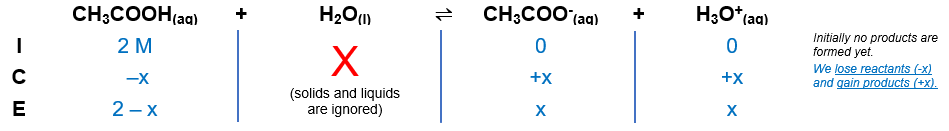# Problem: What is the percent ionization of a 2 M solution of acetic acid at 25 °C? a) 0.6% b) 0.3% c) 0.0036% d) 0.0018%

###### FREE Expert Solution

Recall that the percent ionization is given by:

The dissociation of acetic acid (CH3COOH) is as follows:

CH3COOH(aq) + H2O(l)  H3O+(aq) + A(aq); Ka = 1.8 × 10–5

From this, we can construct an ICE table. Remember that liquids are ignored in the ICE table and Ka expression.The Ka expression for CH3COOH is:

$\overline{){{\mathbf{K}}}_{{\mathbf{a}}}{\mathbf{=}}\frac{\mathbf{products}}{\mathbf{reactants}}{\mathbf{=}}\frac{\mathbf{\left[}{\mathbf{H}}_{\mathbf{3}}{\mathbf{O}}^{\mathbf{+}}\mathbf{\right]}\mathbf{\left[}{\mathbf{CH}}_{\mathbf{3}}{\mathbf{COO}}^{\mathbf{-}}\mathbf{\right]}}{\mathbf{\left[}{\mathbf{CH}}_{\mathbf{3}}\mathbf{COOH}\mathbf{\right]}}}$

${\mathbf{K}}_{\mathbf{a}}\mathbf{=}\frac{\mathbf{\left[}{\mathbf{H}}_{\mathbf{3}}{\mathbf{O}}^{\mathbf{+}}\mathbf{\right]}\mathbf{\left[}{\mathbf{CH}}_{\mathbf{3}}{\mathbf{COO}}^{\mathbf{-}}\mathbf{\right]}}{\mathbf{\left[}{\mathbf{CH}}_{\mathbf{3}}\mathbf{COOH}\mathbf{\right]}}$

$\mathbf{1}\mathbf{.}\mathbf{8}\mathbf{×}{\mathbf{10}}^{\mathbf{-}\mathbf{5}}\mathbf{=}\frac{\mathbf{\left(}\mathbf{x}\mathbf{\right)}\mathbf{\left(}\mathbf{x}\mathbf{\right)}}{\mathbf{2}\mathbf{-}\mathbf{x}}$

$\frac{\mathbf{\left[}{\mathbf{CH}}_{\mathbf{3}}\mathbf{COOH}{\mathbf{\right]}}_{\mathbf{initial}}}{{\mathbf{K}}_{\mathbf{a}}}\gg \mathbf{500}$

Since the ratio is greater than 500, we can remove (–x) from the equation.

The equation simplifies to:

98% (309 ratings)###### Problem Details

What is the percent ionization of a 2 M solution of acetic acid at 25 °C?

a) 0.6%

b) 0.3%

c) 0.0036%

d) 0.0018%

Frequently Asked Questions

What scientific concept do you need to know in order to solve this problem?

Our tutors have indicated that to solve this problem you will need to apply the Weak Acids concept. If you need more Weak Acids practice, you can also practice Weak Acids practice problems.

What professor is this problem relevant for?

Based on our data, we think this problem is relevant for Professor Sparks' class at TEXAS.